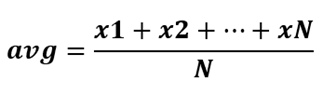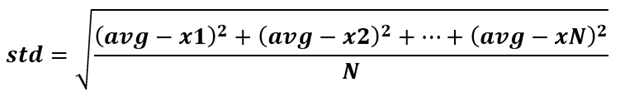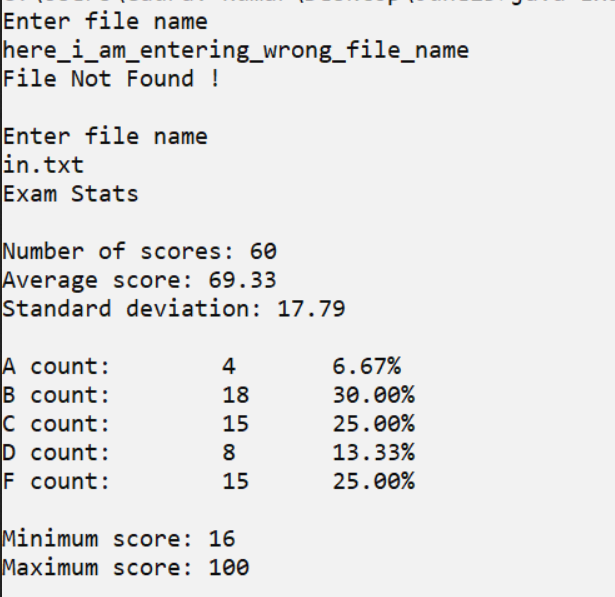## Problem Statement

Design and implement a simple program that processes examination scores from a data file.  The program will determine statistics about the examination, including letter grade counts, and then print the data to the console as well as save the data to a new output file.

## Problem Description

The description is somewhat simple. For this project, you will be writing code to process exam grade data. The processing will include calculating the average, the median, and the standard deviation of the population.  A sample input file features numbers separated by a single white space, like so:

57 80 100 95 55 78 61 53 84 34

69 74 70 74 71 87 26 83 83 79

37 74 85 67 38 82 72 38 74 16

81 81 80 92 82 86 64 56 69 41

81 80 71 59 71 81 77 68 88 67

58 43 75 96 72 81 42 62 75 85

And the output after all data processing should have the format:

Exam Stats

Number of scores: 60

Average score: 69.33

Standard deviation: 17.80

A count:  4    6.67%

B count:  18   30.00%

C count:  15   25.00%

D count:  8    13.33%

F count:  15   25.00%

Minimum score: 16

Maximum score: 100

All decimals numbers must be rounded to two decimals places for this project.

# Project Requirements

Functional Requirements

You will receive an unknown number of exam scores from the input file. A valid score must be between 0 and 100. If a number is larger than 100 or smaller than 0, that number should be ignored and not included in the exam stats.

The scores must be used to calculate the exam stats and print them to the output file.

The program must request the input filename from the user through the console. If the file doesn’t exist, the user should be informed and prompted again until they give one that does exist.

The output must contain:

o   The total exam count (not including bad data, like negatives or outside range)

o   The average score

o   The population standard deviation of the scores

o   The number and percentage of scores for each letter grade group

o   The minimum score

o   The maximum score

As mentioned, all decimals must be rounded to two decimal places

The following formulas are needed for this project:

o   Average: The average score is the sum of the individual scores divided by the total number of scores. Avoid integer division. If N is the total number of scores and x1, x2, …, xN are the scores (and avg is abbreviation for average), then

..

Population standard deviation: The difference of each score and the average squared, added, divided by N, and take the square root of the result. Given the notation from the average formula (and std is abbreviation for standard deviation), then...

### Technical Requirements

You must use constant variables to store the lower score limits for letter grade groups.  90, 80, 70, and 60 coincide to the lower bounds for grades A, B, C, D.

For these constants, declare them above the main method (but still inside the class brackets) as static final variables – this is a new concept, called a class variable

You will need variables to hold data such as minimumScore, maximumScore, totalCount, count of various grades, etc.

Make sure you use a Scanner object and File object for reading the file

You also need a Scanner object for getting the file name from the user through the console.

Make sure to use a while loop to process the text file – determining what goes inside the while loop is up to you. After the while loop, you should calculate the average

Then, you must read the file again to calculate the standard deviation! The reason for this is you need to calculate the (average – score) for every score! This second while loop will be similar to the first one.

After all the calculation is finished, output to the file and the console.

### Documentation Requirements

Place a comment above the class, using /** style comments */, that includes your name and briefly describes the project

Put a comment in your code before every major block of the problem – it is up to you what you consider major enough to comment on

Below are a few more samples of data to test

Sample 1 Scores

31 87 26 83 83 79 37 74 85 67

82 72 38 74 16 81 81 80 92 82

86 64 56 69 41 81 71 59 71 81

77 68 88 67 58 43 75 96 72 81

42 62 75 85 90 100 94 80 80 80

60 65 40 72 63 52 74 85 74 85

24 48

Sample 1 Output

Exam Stats

Number of scores: 62

Average score: 69.10

Standard deviation: 18.87

A count:  5    8.06%

B count:  20   32.26%

C count:  13   20.97%

D count:  9    14.52%

F count:  15   24.19%

Minimum score: 16

Maximum score: 100

Sample 2 Scores

110 90 90 95 95 60 75 71 92 88

100 -90

Sample 2 Output

Exam Stats

Number of scores: 10

Average score: 85.6

Standard deviation: 12.04

A count:  6    60.00%

B count:  1    10.00%

C count:  2    20.00%

D count:  1    10.00%

F count:  0    0.00%

Minimum score: 60

Maximum score: 100

## Hand-In

Submit your zipped project folder to Blackboard.

## Evaluation

Correctness (80 points).  These points will be allocated as follows:

5 points for correct constant set up

10 points for correct file solicitation and opening

25 points for correctly performing the first while loop and getting the average, min, max

15 points for correctly performing the second while loop and getting the std

25 points for the correct output report

Design (10 points). These points will be allocated based on data fields, methods, classes, and algorithms used to complete the design of the required classes.

Documentation and Style (10 points): Your code should have a clean, professional appearance.  Remember to put your name and the project name at the top of all code files

import java.io.File;
import java.io.FileInputStream;
import java.io.FileNotFoundException;
import java.io.PrintWriter;
import java.util.Scanner;

public class ExamStats {

static String fileName;

public static final int LOWER_LIMIT_FOR_GRADE_A = 90;
public static final int LOWER_LIMIT_FOR_GRADE_B = 80;
public static final int LOWER_LIMIT_FOR_GRADE_C = 70;
public static final int LOWER_LIMIT_FOR_GRADE_D = 60;

public static void main(String[] args) throws Exception {

// Declaring variables
int totalExamCount = 0, totalExamScore = 0, minScore = 101, maxScore = -1;
double avgScore, standardDeviation;

// 'fileScanner' for reading from file and 'out' for writing into file out.txt
Scanner fileScanner = getFileScanner();
PrintWriter out = new PrintWriter("out.txt");

// First while loop for calculating average and other required data
while(fileScanner.hasNextInt()) {
int currScore = fileScanner.nextInt();
if(currScore >= 0 && currScore <= 100) {
totalExamCount += 1;
totalExamScore += currScore;
minScore = Math.min(minScore, currScore);
maxScore = Math.max(maxScore, currScore);

}
}

avgScore = (double) totalExamScore / totalExamCount;

try {fileScanner = new Scanner(new File(fileName));} catch (Exception e) {}

long numeraturForDeviation = 0;

// Second while loop for calculating Standard Deviation
while(fileScanner.hasNextInt()) {
int currScore = fileScanner.nextInt();
if(currScore >= 0 && currScore <= 100) {
double temp = (avgScore - currScore) * (avgScore - currScore);
numeraturForDeviation += temp;
}
}

standardDeviation = Math.sqrt((double)numeraturForDeviation / totalExamCount);

// Writing data into console
System.out.println("Exam Stats\n");
System.out.println("Number of scores: " + totalExamCount);
System.out.printf("Average score: %.2f\n", avgScore);
System.out.printf("Standard deviation: %.2f\n\n", standardDeviation);
System.out.println("\nMinimum score: " + minScore);
System.out.println("Maximum score: " + maxScore);

// Writing data into file out.txt
out.println("Exam Stats\n");
out.println("Number of scores: " + totalExamCount);
out.printf("Average score: %.2f\n", avgScore);
out.printf("Standard deviation: %.2f\n\n", standardDeviation);
out.println("\nMinimum score: " + minScore);
out.println("Maximum score: " + maxScore);

// colsing resorrces
fileScanner.close();
out.close();
}

// getFileScanner will return the Scnnaer object for reading data from file
public static Scanner getFileScanner () {
Scanner scResult = null;
Scanner sc = new Scanner(System.in);
try {
System.out.println("Enter file name");
fileName = sc.next();
File file = new File(fileName);
scResult = new Scanner(file);
} catch (Exception e) {
return getFileScanner();
}
sc.close();
return scResult;
}
}

OUTPUT:Share: